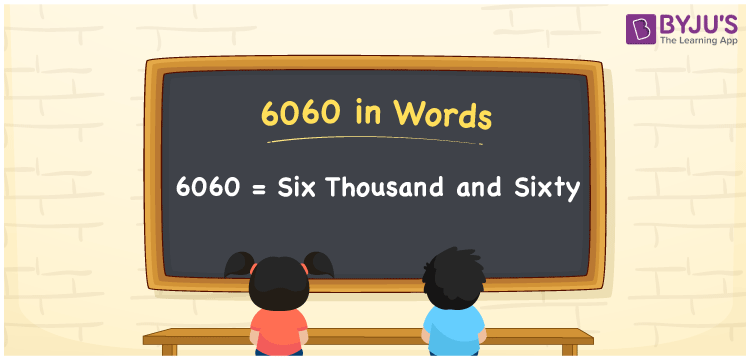# 6060 in Words

The number name of 6060 is Six thousand and sixty. The number 6060 is a cardinal number, and it is a four-digit number. For example, If you spent Rs. 6060 on purchasing clothes, you can write it as “I spent Rs. Six thousand and sixty on purchasing clothes”. We know that the place value system is used to write the number 6060 in words. In this article, let us discuss the spelling of 6060 in English words and its procedure in detail.

 6060 in Words: Six Thousand and Sixty. Six Thousand and Sixty in Numerical Form: 6060.

## 6060 in English Words## How to Write 6060 in Words?

The place values of 6060 are provided below:

 Thousands Hundreds Tens Ones 6 0 6 0

The expanded form of 6060 is as follows:

= 6 × Thousand + 0 × Hundred + 6 × Ten + 0 × One

= 6 × 1000 + 0 × 100 + 6 × 10 + 0 × 1

= 6000 + 60

= 6060

= Six thousand and sixty

Hence, 6060 in words is six thousand and sixty.

6060 in words – Six thousand and sixty

Is 6060 an odd number? – No

Is 6060 an even number? – Yes

Is 6060 a perfect square number? – No

Is 6060 a perfect cube number? – No

Is 6060 a prime number? – No

Is 6060 a composite number? – Yes

## Frequently Asked Questions on 6060 in Words

Q1

### How to spell 6060 in English words?

6060 in words is six thousand and sixty.

Q2

### Simplify 6000 + 60, and express it in words.

Simplifying 6000 + 60, we get 6060. Hence, 6060 in words is six thousand and sixty.

Q3

### How to write six thousand and sixty in numbers?

Six thousand and sixty in numbers is 6060.